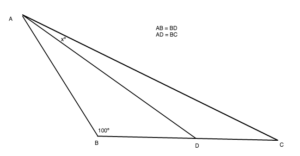Ask Uncle Colin is a chance to ask your burning, possibly embarrassing, maths questions – and to show off your skills at coming up with clever acronyms. Send your questions to colin@flyingcoloursmaths.co.uk and Uncle Colin will do what he can.

Dear Uncle Colin,
I’ve been given a trigonometry problem I can’t make head or tail of!

In this triangle, AB=BD and AD=BC, while angle ABD = 100º. What’s angle DAC?What on earth am I supposed to do?

Extremely Unhappy, Confused Learner In Difficulties

Hello, EUCLID, thanks for your message. That’s a tricky one! I have a solution, but I don’t like it much! It relies on the sine rule and a few trigonometric identities.

Looking at the leftmost triangle, it’s clear that the angle at the top (BAD) is the same as the angle at the bottom right (ADB), and they both have to be 40º. The sine rule says $\frac{AB}{\sin(40º)} = \frac{AD}{\sin(100º)}$. That can be rearranged to give $\frac{AB}{AD} = \frac{\sin(40º)}{\sin(100º)}$.

Looking at the whole triangle, you can see that the top angle (BAC) is $(40+x)º$ and the bottom right (ACB) is $(40-x)º$, meaning that $\frac{AB}{\sin(40-x)º} = \frac{BC}{\sin(40+x)º}$ or, better, $\frac{AB}{BC} = \frac{\sin(40-x)º}{\sin(40+x)º}$.

Because $AC = BD$, these two fractions are the same, meaning $\frac{\sin(40º)}{\sin(100º)} = \frac{\sin(40-x)º}{\sin(40+x)º}$.

This has been, in the words of another student, hit up the denominator with the ugly stick. But wait: it gets worse! Let’s expand the right-hand side: $\frac{\sin(40º)}{\sin(100º)} = \frac{\sin(40º)cos(xº)-\cos(40º)\sin(xº)}{\sin(40º)cos(xº)+\sin(xº)\cos(40º)}$ … and divide the top and bottom of it by $\cos(xº)cos(40º)$ to make it:

$\frac{\sin(40º)}{\sin(100º)} = \frac{\tan(40º)-\tan(xº)}{\tan(40º)+\tan(xº)}$ Another trick we can use: on the left, $\sin(100º) = \sin(80º) = 2\sin(40º)cos(40º)$, using double-angle formulas. That means the left-hand side is $\frac{1}{2\cos(40º)}$. I’m going to introduce some letters to save typing, now: let $t = \tan(xº)$, $T = \tan(40º)$, and $c = \cos(40º)$. The equation is now $\frac{1}{2C} = \frac{T-t}{T+t}$, which can be rearranged as: $T + t = 2C(T-t)$ or: $t(2C+1) = T(2C - 1)$ which means: $t = T\frac{2C - 1}{2C+1}$ Aha! We have $t$ on its own. Let’s go back to the angles: $\tan(xº) = \tan(40º) \frac{ 2 \cos(40º) - 1}{2\cos(40º) + 1}$ If you were so inclined, you could put this into the calculator and work out $x$. However, there’s a cleverer way ((by ‘clever’, I mean ‘show-off’)): we can get the exact answer using the ‘formulas you never use’ in the C3 section of the formula book. Also, remember that $\cos(60º) = \frac 12$. $\tan(x) = \tan(40º) \frac{ 2 \cos(40º) - 2 \cos(60º) }{2 \cos(40º) + 2 \cos(60º)}$ Divide top and bottom by 2, and apply $\cos(A) - \cos(B) \equiv -2\sin\left(\frac{A+B}{2}\right) \sin\left(\frac{A-B}{2}\right)$ and $\cos(A) + \cos(B) \equiv 2\cos\left(\frac{A+B}{2}\right) \cos\left(\frac{A-B}{2}\right)$ to get: $\tan(x) = \tan(40º) \frac{ -2 \sin(50º) \sin(-10º) }{2 \cos(50º) \cos(-10º)}$ Being smart with the signs gives: $\tan(x) = \tan(40º) tan(50º) \tan (10º)$ And since $\tan(40º) \tan(50º) = 1$, $\tan(xº) = \tan(10º)$ Phew! I’m not surprised you found it tricky – and I wouldn’t be surprised to learn there’s an easier way. – Uncle Colin

* Edited 2015-09-26 to fix HTML